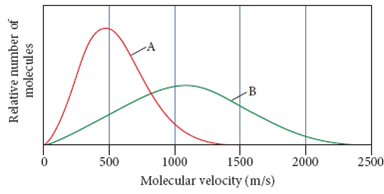# Problem: This graph shows the distribution of molecular velocities for two different molecules (A and B) at the same temperature. Which molecule has the higher molar mass? Which molecule would have the higher rate of effusion?

###### FREE Expert Solution

Recall that

• As the molecular weight increase, the molecules moving at higher velocity decrease.
• As the molecular weight decrease, the curve gets broader and lower.

94% (143 ratings)###### Problem Details

This graph shows the distribution of molecular velocities for two different molecules (A and B) at the same temperature. Which molecule has the higher molar mass? Which molecule would have the higher rate of effusion?